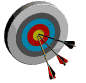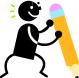# Intervention Strategies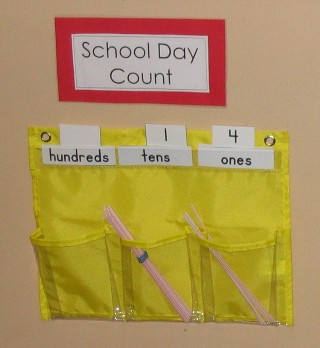## Featured Topic: Understanding and Using Place Value (Tens and Ones)

Students need lots of experiences associating the digits in a two-digit number with the number of tens and ones. Rote counting does not develop this important base-ten conceptual understanding. Students must decompose the numbers and represent them with grouped materials to develop the concept of our system of tens and ones.

This conceptual understanding of the base-ten system is critical as students move to larger numbers that are not easily represented by concrete materials. They will extend the concept to larger numbers and build understanding on that. Hence, it is important that young students develop a meaningful understanding of this representation.

Suggested Strategies:

• School Day Count: In addition to adding a straw and grouping materials in cups for hundreds-tens-ones, use number cards or a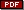School Day Count dry-erase sheet so that students write the numerals in the correct base-ten notation over these boxes or cups to show how many ones, how many tens, how many hundreds. This builds the sense of place value through daily practice and reflection about what part of the number changes when you add one more straw or one more group of ten straws, etc.
• Groupable Materials: Begin with groupable materials (e.g. straws, popsicle sticks, snap cubes) so that the student is able to build a sense of tens by grouping the materials by tens.
• Money: NOTE: pennies and dimes are not appropriate materials for building place value as the student is not able to group pennies into a dime or break a dime into pennies. Also, if you think about it, the dime is smaller than one penny so this makes no sense to a student trying to develop a sense of ten as a larger number. Money concepts and skills are built separately.
• Linking Cubes: Use a place value workmat for snapcubes. The student places one cube in each space. When he/she has filled all ten spaces, the student snaps the cubes together and moves the cube train or rod into the tens column of the workmat.
• DownloadLinking Cube Mat.
• Number Stories: When the student is proficient at expressing numbers using groupable materials, introduce number stories and let the student add cubes to the mat for each addend. He/she should regroup as necessary and use the final result to answer the problem.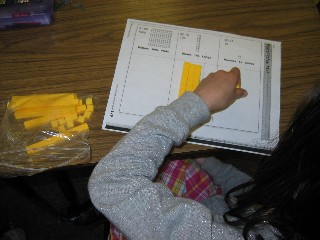• Transition to Pre-grouped Materials: When the student is proficient at working with groupable materials, introduce the base ten blocks and begin using these in a comfortable format for that student (e.g. showing numbers on the snap cube workmat, solving simple addition and subtraction stories, etc.). When the student understands that ten cubes trade for a rod and does this without prompting, introduce theplain tens-one mat and continue practice. You may need to provide some prompting initially but the student needs to be actively watching the ones section of the mat.
• Number Cards: Introducedigit cards so that the student is able to first express the number using rods and cubes, then select the correct number cards to place in the ones and tens section to name that number and show the number of tens and ones in the number. As an alternative to digit cards, if mats are inserted into sheet protectors, students may write the digits at the top of each column to represent the number.• Hundred Chart or Number Grid: Monitor student use of place value cues in thenumber grid. Students who fail to develop working knowledge of the patterns, need to build the hundred grid withsmall number cards or tiles to recognize the patterns and develop this sense of place value patterns in the number grid. Provide models at center time and build row by row as the student becomes proficient at quickly assembling random numbers, using place value cues.
• Hundred Chart Puzzles Copyhundred chart on card stock, laminate and cut apart to form hundred chart puzzles. Differentiate the number of pieces and the intricacy of the pieces to create developmentally challenging versions of this activity. Place the pieces in a baggie and mark the baggies to reflect level of difficulty (e.g. 1-5 apples, stars, etc.). Place the puzzles in the math center for student use.

## Games for Practice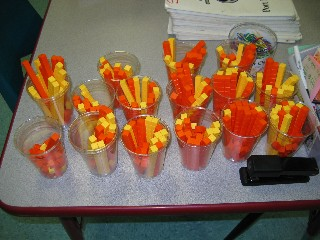### Big Number

Students use a deck ofnumber cards. Teachers may differentiate the size of each deck to meet the developmental needs of learners in the class. Each player selects a card from the pile and places it in front of him/her. He/she then uses base ten blocks to build the number on the place value mat. When this is complete, players decide who has the largest number for that round. This player wins a chip. Players discard their numbers, clear their mats, and select new cards for the next round.

• Downloadnumber cards
• DownloadPlace Value Mat,

### Extensions for Big Number Game:

• Hundred Board Play: each student uses a different color marker in this version of the Big Number game. Each student picks a card from the top of the deck, and places his/her marker on that number on theHundred Board. When all markers have been placed, the students decide who had the biggest number for that round. That player wins a point. Students then discard number cards, clear the hundred board and select new cards for the next round.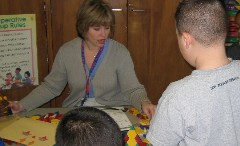## Mathwire.com Intervention Strategies Resources